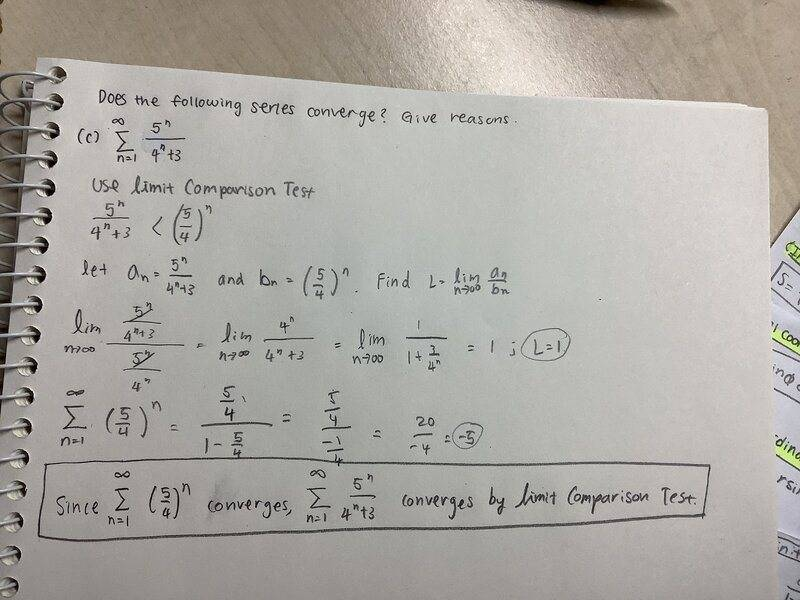# Determining if a series converges

• Sunwoo Bae

#### Sunwoo Bae

Homework Statement
Does the following series converge? Give reasons. (Series shown below)
Relevant Equations
NoneThe following is my attempt at the solution.
Here, I used limit comparison test to arrive at the answer that the series converges.
I am confused because I cannot figure where my work went wrong…
can anyone tell me how the series diverges, and why my work is incorrect?

Thank you!

The geometric series with terms ##(\frac 5 4)^n## clearly diverges. The formula for a convergent geometric series does not apply. And, in particular, the sum of the series in not ##-5##. That would be too absurd!

The geometric series with terms ##(\frac 5 4)^n## clearly diverges. The formula for a convergent geometric series does not apply. And, in particular, the sum of the series in not ##-5##. That would be too absurd!
Got it! Thank you!

•berkeman
That would be too absurd!
As opposed to just absurd enough?Homework Statement:: Does the following series converge? Give reasons. (Series shown below)
Relevant Equations:: None